### 2 CART算法

#### 2.1 分类树生成算法

$\text{Gini}\left(p\right)=\sum _{k=1}^{K}{p}_{k}\left(1-{p}_{k}\right)=1-\sum _{k=1}^{K}{p}_{k}^{2}\phantom{\rule{0.278em}{0ex}}\phantom{\rule{0.278em}{0ex}}\phantom{\rule{0.278em}{0ex}}\phantom{\rule{0.278em}{0ex}}\phantom{\rule{0.278em}{0ex}}\phantom{\rule{0.278em}{0ex}}\phantom{\rule{0.278em}{0ex}}\left(1\right)$

$\text{Gini}\left(D\right)=1-\sum _{k=1}^{K}{\left(\frac{|{C}_{k}|}{|D|}\right)}^{2}\phantom{\rule{0.278em}{0ex}}\phantom{\rule{0.278em}{0ex}}\phantom{\rule{0.278em}{0ex}}\phantom{\rule{0.278em}{0ex}}\phantom{\rule{0.278em}{0ex}}\phantom{\rule{0.278em}{0ex}}\phantom{\rule{0.278em}{0ex}}\left(2\right)$

${D}_{1}=\left\{\left(x,y\right)\in D|A\left(x\right)=a\right\},{D}_{2}=D-{D}_{1}\phantom{\rule{0.278em}{0ex}}\phantom{\rule{0.278em}{0ex}}\phantom{\rule{0.278em}{0ex}}\phantom{\rule{0.278em}{0ex}}\phantom{\rule{0.278em}{0ex}}\phantom{\rule{0.278em}{0ex}}\phantom{\rule{0.278em}{0ex}}\left(3\right)$

$\text{Gini}\left(D,A\right)=\frac{|{D}_{1}|}{|D|}\text{Gini}\left({D}_{1}\right)+\frac{|{D}_{2}|}{|D|}\text{Gini}\left({D}_{2}\right)\phantom{\rule{0.278em}{0ex}}\phantom{\rule{0.278em}{0ex}}\phantom{\rule{0.278em}{0ex}}\phantom{\rule{0.278em}{0ex}}\phantom{\rule{0.278em}{0ex}}\phantom{\rule{0.278em}{0ex}}\phantom{\rule{0.278em}{0ex}}\left(4\right)$

(1) 设训练集为$D$$D$，根据式$\left(2\right)$$(2)$计算现有特征对该数据集的基尼不纯度。接着，对于每一个特征$A$$A$，对其可能的每一个值$a$$a$，根据样本点对$A=a$$A=a$是否成立将$D$$D$划分成${D}_{1},{D}_{2}$$D_1,D_2$两个部分，然后再利用式$\left(4\right)$$(4)$计算$A=a$$A=a$时的基尼不纯度；

(2) 在所有可能的特征$A$$A$以及它们所有可能的切分点$a$$a$中，选择基尼不纯度最小的特征取值作为划分标准将原有数据集划分为两个部分，并分配到两个子节点中去；

(3) 对两个子节点递归的调用步骤(1)和(2)，直到满足停止条件；

(4) 生成CART决策树。

#### 2.2 分类树生成示例

$\text{Gini}\left(D\right)=1-\sum _{k=1}^{K}{\left(\frac{|{C}_{k}|}{|D|}\right)}^{2}=1-{\left(\frac{5}{15}\right)}^{2}-{\left(\frac{10}{15}\right)}^{2}\approx 0.444\phantom{\rule{0.278em}{0ex}}\phantom{\rule{0.278em}{0ex}}\phantom{\rule{0.278em}{0ex}}\phantom{\rule{0.278em}{0ex}}\phantom{\rule{0.278em}{0ex}}\phantom{\rule{0.278em}{0ex}}\phantom{\rule{0.278em}{0ex}}\left(5\right)$

$\text{Gini}\left(D,{A}_{1}=1\right)=\frac{7}{15}\text{Gini}\left({D}_{1}\right)+\frac{8}{15}\text{Gini}\left({D}_{2}\right)=\frac{7}{15}×\frac{24}{49}+\frac{8}{15}×\frac{14}{64}\approx 0.345\phantom{\rule{0.278em}{0ex}}\phantom{\rule{0.278em}{0ex}}\phantom{\rule{0.278em}{0ex}}\phantom{\rule{0.278em}{0ex}}\phantom{\rule{0.278em}{0ex}}\phantom{\rule{0.278em}{0ex}}\phantom{\rule{0.278em}{0ex}}\left(6\right)$

$\text{Gini}\left(D,{A}_{2}=1\right)=\frac{8}{15}\text{Gini}\left({D}_{1}\right)+\frac{7}{15}\text{Gini}\left({D}_{2}\right)=\frac{8}{15}×\frac{1}{2}+\frac{7}{15}×\frac{12}{49}\approx 0.381\phantom{\rule{0.278em}{0ex}}\phantom{\rule{0.278em}{0ex}}\phantom{\rule{0.278em}{0ex}}\phantom{\rule{0.278em}{0ex}}\phantom{\rule{0.278em}{0ex}}\phantom{\rule{0.278em}{0ex}}\phantom{\rule{0.278em}{0ex}}\left(6\right)$

$\text{Gini}\left(D,{A}_{3}=D\right)=\frac{12}{15}\text{Gini}\left({D}_{1}\right)+\frac{3}{15}\text{Gini}\left({D}_{2}\right)=\frac{12}{15}×\frac{4}{9}+\frac{3}{15}×\frac{4}{9}\approx 0.444\phantom{\rule{0.278em}{0ex}}\phantom{\rule{0.278em}{0ex}}\phantom{\rule{0.278em}{0ex}}\phantom{\rule{0.278em}{0ex}}\phantom{\rule{0.278em}{0ex}}\phantom{\rule{0.278em}{0ex}}\phantom{\rule{0.278em}{0ex}}\left(8\right)$
$\text{Gini}\left(D,{A}_{3}=S\right)=\frac{11}{15}\text{Gini}\left({D}_{1}\right)+\frac{4}{15}\text{Gini}\left({D}_{2}\right)=\frac{11}{15}×\frac{56}{121}+\frac{4}{15}×\frac{3}{8}\approx 0.439\phantom{\rule{0.278em}{0ex}}\phantom{\rule{0.278em}{0ex}}\phantom{\rule{0.278em}{0ex}}\phantom{\rule{0.278em}{0ex}}\phantom{\rule{0.278em}{0ex}}\phantom{\rule{0.278em}{0ex}}\phantom{\rule{0.278em}{0ex}}\left(9\right)$
$\text{Gini}\left(D,{A}_{3}=T\right)=\frac{7}{15}\text{Gini}\left({D}_{1}\right)+\frac{8}{15}\text{Gini}\left({D}_{2}\right)=\frac{7}{15}×\frac{20}{49}+\frac{8}{15}×\frac{30}{64}\approx 0.440\phantom{\rule{0.278em}{0ex}}\phantom{\rule{0.278em}{0ex}}\phantom{\rule{0.278em}{0ex}}\phantom{\rule{0.278em}{0ex}}\phantom{\rule{0.278em}{0ex}}\phantom{\rule{0.278em}{0ex}}\phantom{\rule{0.278em}{0ex}}\left(10\right)$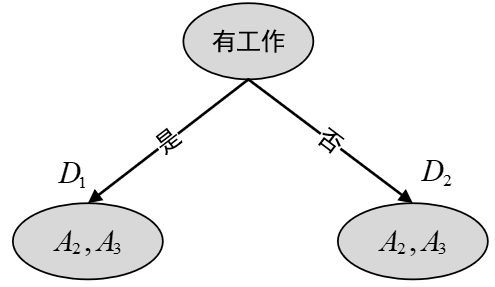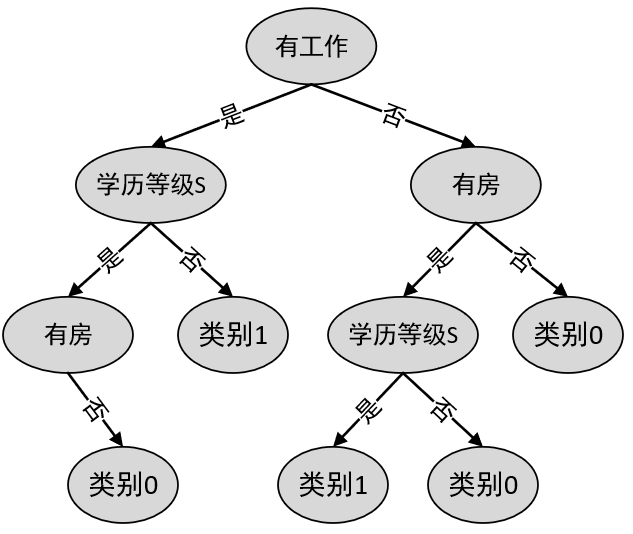#### 2.3 分类树剪枝步骤

(1) 首先是从之前生成的决策树${T}_{0}$$T_0$底端开始不断剪枝，直到${T}_{0}$$T_0$的根节点，形成一个子序列$\left\{{T}_{0},{T}_{1},...,{T}_{n}\right\}$$\{T_0,T_1,...,T_n\}$

(2) 然后通过交叉验证对这一子序列进行测试，从中选择最优的子树。

• 剪枝，形成一个子序列

在剪枝过程中，计算子树的损失函数

${C}_{\alpha }\left(T\right)=C\left(T\right)+\alpha |T|\phantom{\rule{0.278em}{0ex}}\phantom{\rule{0.278em}{0ex}}\phantom{\rule{0.278em}{0ex}}\phantom{\rule{0.278em}{0ex}}\phantom{\rule{0.278em}{0ex}}\phantom{\rule{0.278em}{0ex}}\phantom{\rule{0.278em}{0ex}}\left(11\right)$

其中，$T$$T$为任意子树，$C\left(T\right)$$C(T)$为对训练集的预测误差，$|T|$$|T|$为子树的叶节点个数，$\alpha \ge 0$$\alpha\geq0$为参数。需要指出的是不同与之前ID3和C4.5中剪枝算法的$\alpha$$\alpha$，前者是人为设定，而此处则是通过计算得到。

具体地，从整体树${T}_{0}$$T_0$开始剪枝，如图3所示。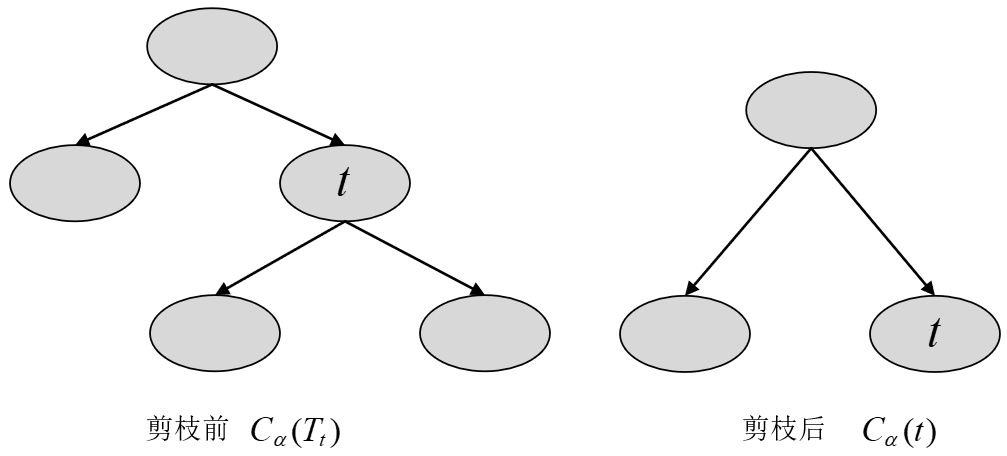图 3. CART剪枝图

对于${T}_{0}$$T_0$的任意内部节点$t$$t$，以$t$$t$为根节点的子树${T}_{t}$$T_t$（可以看作是剪枝前）的损失函数为

${C}_{\alpha }\left({T}_{t}\right)=C\left({T}_{t}\right)+\alpha |{T}_{t}|\phantom{\rule{0.278em}{0ex}}\phantom{\rule{0.278em}{0ex}}\phantom{\rule{0.278em}{0ex}}\phantom{\rule{0.278em}{0ex}}\phantom{\rule{0.278em}{0ex}}\phantom{\rule{0.278em}{0ex}}\phantom{\rule{0.278em}{0ex}}\left(12\right)$

同时，以$t$$t$为单节点的子树（可以看作是剪枝后）的损失函数为

${C}_{\alpha }\left(t\right)=C\left(t\right)+\alpha \cdot 1\phantom{\rule{0.278em}{0ex}}\phantom{\rule{0.278em}{0ex}}\phantom{\rule{0.278em}{0ex}}\phantom{\rule{0.278em}{0ex}}\phantom{\rule{0.278em}{0ex}}\phantom{\rule{0.278em}{0ex}}\phantom{\rule{0.278em}{0ex}}\left(13\right)$

① 当$\alpha =0$$\alpha=0$或者极小的时候，有不等式

${C}_{\alpha }\left({T}_{t}\right)<{C}_{\alpha }\left(t\right)\phantom{\rule{0.278em}{0ex}}\phantom{\rule{0.278em}{0ex}}\phantom{\rule{0.278em}{0ex}}\phantom{\rule{0.278em}{0ex}}\phantom{\rule{0.278em}{0ex}}\phantom{\rule{0.278em}{0ex}}\phantom{\rule{0.278em}{0ex}}\left(14\right)$

不等式成立的原因是因为，当$\alpha =0$$\alpha=0$或者极小的时候，起决定作用的就是预测误差$C\left(t\right)$$C(t)$$C\left({T}_{t}\right)$$C(T_t)$，而模型越复杂其训练误差总是越小的，因此不等式成立。

② 当$\alpha$$\alpha$增大时，在某一$\alpha$$\alpha$

${C}_{\alpha }\left({T}_{t}\right)={C}_{\alpha }\left(t\right)\phantom{\rule{0.278em}{0ex}}\phantom{\rule{0.278em}{0ex}}\phantom{\rule{0.278em}{0ex}}\phantom{\rule{0.278em}{0ex}}\phantom{\rule{0.278em}{0ex}}\phantom{\rule{0.278em}{0ex}}\phantom{\rule{0.278em}{0ex}}\left(15\right)$

等式成立的原因是因为，当$\alpha$$\alpha$慢慢增大时，就不能忽略模型复杂度所带来的影响（也就是式$\left(11\right)$$(11)$第二项）。所以总会存在一个取值$\alpha$$\alpha$使得等式$\left(15\right)$$(15)$成立。

③ 当$\alpha$$\alpha$再增大时，不等式$\left(14\right)$$(14)$反向

因此，当${C}_{\alpha }\left({T}_{t}\right)={C}_{\alpha }\left(t\right)$$C_{\alpha}(T_t)=C_{\alpha}(t)$时，有$\alpha =\frac{C\left(t\right)-C\left({T}_{t}\right)}{|{T}_{t}|-1}$$\alpha=\frac{C(t)-C(T_t)}{|T_t|-1}$，此时的子树${T}_{t}$$T_t$和单节点树$t$$t$有相同的损失函数值，但$t$$t$的节点少模型更简单，因此$t$$t$${T}_{t}$$T_t$更可取，即对${T}_{t}$$T_t$进行剪枝。

为此，对决策树${T}_{0}$$T_0$中每一个内部节点$t$$t$来说，都可以计算

$g\left(t\right)=\frac{C\left(t\right)-C\left({T}_{t}\right)}{|{T}_{t}|-1}\phantom{\rule{0.278em}{0ex}}\phantom{\rule{0.278em}{0ex}}\phantom{\rule{0.278em}{0ex}}\phantom{\rule{0.278em}{0ex}}\phantom{\rule{0.278em}{0ex}}\phantom{\rule{0.278em}{0ex}}\phantom{\rule{0.278em}{0ex}}\left(16\right)$

它表示剪枝后整体损失函数减少的程度。因为每个$g\left(t\right)$$g(t)$背后都对应着一个决策树模型，而不同的$g\left(t\right)$$g(t)$则表示损失函数变化的不同程度。接着，在树${T}_{0}$$T_0$中减去$g\left(t\right)$$g(t)$最小的子树${T}_{t}$$T_t$，将得到的子树作为${T}_{1}$$T_1$。如此剪枝下去，直到得到根节点。

注意，此时得到的一系列$g\left(t\right)$$g(t)$$\alpha$$\alpha$，都能使得在每种情况下剪枝前和剪枝后的损失值相等，因此按照上面第②种情况中的规则要进行剪枝。但为什么是减去其中$g\left(t\right)$$g(t)$最小的呢？

对于树$T$$T$来说，其内部可能的节点$t$$t$${t}_{0},{t}_{1},{t}_{2},{t}_{3}$$t_0,t_1,t_2,t_3$${t}_{i}$$t_i$表示其中任意一个, 如图4所示。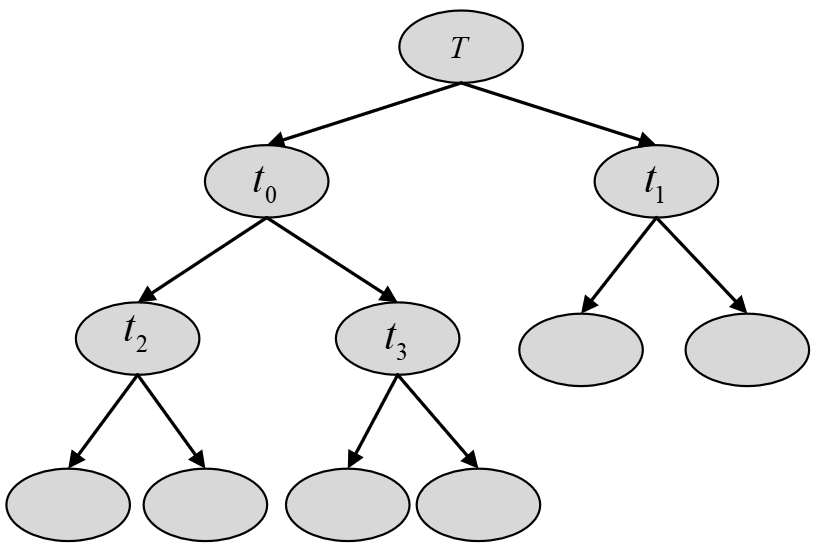图 4. CART剪枝过程图

因此便可以计算得到$g\left({t}_{0}\right),g\left({t}_{1}\right),g\left({t}_{2}\right),g\left({t}_{3}\right)$$g(t_0),g(t_1),g(t_2),g(t_3)$，也即对应的${\alpha }_{0},{\alpha }_{1},{\alpha }_{2},{\alpha }_{3}$$\alpha_0,\alpha_1,\alpha_2,\alpha_3$。从上面的第②种情况可以知道，$g\left(t\right)$$g(t)$是根据式$\left(16\right)$$(16)$所计算得到，因此这4种情况下${t}_{i}$$t_i$${T}_{{t}_{i}}$$T_{t_i}$更可取，都满足剪枝。但是由于以${t}_{i}$$t_i$为根节点的子树对应的复杂度各不相同，也就意味着${\alpha }_{i}\ne {\alpha }_{j},\left(i,j=0,1,2,3;i\ne j\right)$$\alpha_i\neq\alpha_j,(i,j=0,1,2,3;i\neq j)$,即${\alpha }_{i},{\alpha }_{j}$$\alpha_i,\alpha_j$存在着大小关系。又因为，当$\alpha$$\alpha$大的时候，最优子树${T}_{\alpha }$$T_{\alpha}$偏小；当$\alpha$$\alpha$小的时候，最优子树${T}_{\alpha }$$T_{\alpha}$偏大；并且由于在这4中情况下剪枝前和剪枝后损失值都相等（即都满足剪枝条件），因此选择减去其中$g\left(t\right)$$g(t)$最小的子树。

接着，在得到子树${T}_{1}$$T_1$后，再通过上述步骤对${T}_{1}$$T_1$进行剪枝得到${T}_{2}$$T_2$。如此剪枝下去直到得到根节点，此时便得到了子树序列${T}_{0},{T}_{1},{T}_{2},....{T}_{n}$$T_0,T_1,T_2,....T_n$

• 交叉验证选择最优子树${T}_{\alpha }$$T_{\alpha}$

通过上一步步我们便可以得到一系列的子树序列${T}_{0},{T}_{1},...,{T}_{n}$$T_0,T_1,...,T_n$，最后再通过交叉验证来选取最优决的策树${T}_{\alpha }$$T_{\alpha}$

(1) 设$k=0,T={T}_{0}$$k=0,T=T_0$;

(2) 设$\alpha =+\mathrm{\infty }$$\alpha =+\infty$;

(3) 对于树$T$$T$来说，自下而上地对其每个内部节点$t$$t$计算$C\left({T}_{t}\right),|{T}_{t}|$$C({{T}_{t}}),|{{T}_{t}}|$以及

$g\left(t\right)=\frac{C\left(t\right)-C\left({T}_{t}\right)}{|{T}_{t}|-1},\alpha =min\left(\alpha ,g\left(t\right)\right)\phantom{\rule{0.278em}{0ex}}\phantom{\rule{0.278em}{0ex}}\phantom{\rule{0.278em}{0ex}}\phantom{\rule{0.278em}{0ex}}\phantom{\rule{0.278em}{0ex}}\phantom{\rule{0.278em}{0ex}}\phantom{\rule{0.278em}{0ex}}\left(17\right)$

(4) 对$g\left(t\right)=\alpha$$g(t)=\alpha$对应的内部节点$t$$t$进行剪枝，并对叶节点以多数表决法决定其类别得到树$T$$T$

(5) 令$k=k+1$$k=k+1$${\alpha }_{k}=\alpha$$\alpha_k=\alpha$${T}_{k}=T$$T_k=T$

(6) 如果${T}_{k}$$T_k$不是由根节点及两个叶节点构成的树，则继续执行步骤(3)；否则令${T}_{k}={T}_{n}$$T_k=T_n$

(7) 采用交叉验证在子树序列${T}_{0},{T}_{1},...,{T}_{n}$${{T}_{0}},{{T}_{1}},...,{{T}_{n}}$中选择最优子树${T}_{\alpha }$$T_{\alpha}$

#### 2.4 分类树剪枝示例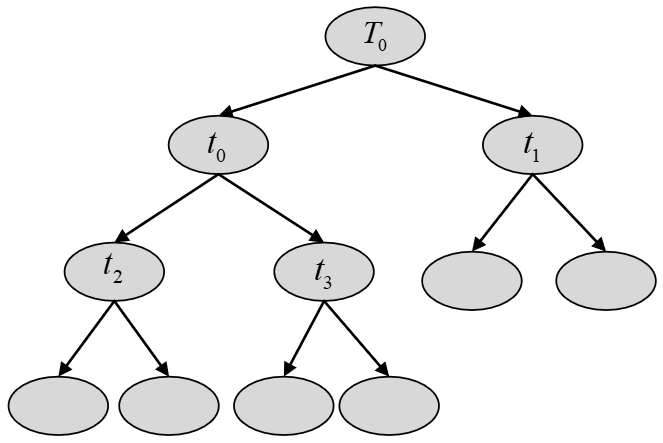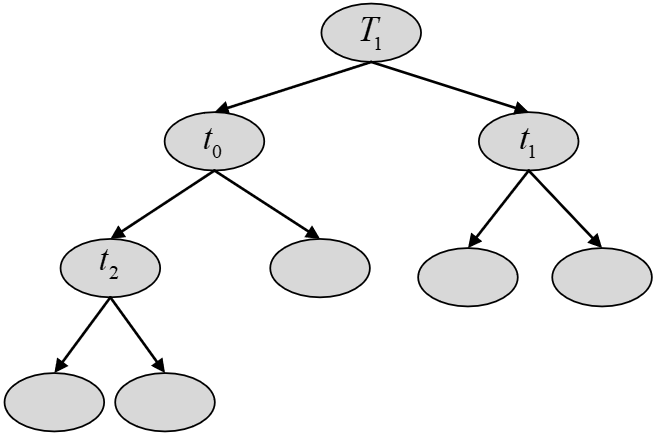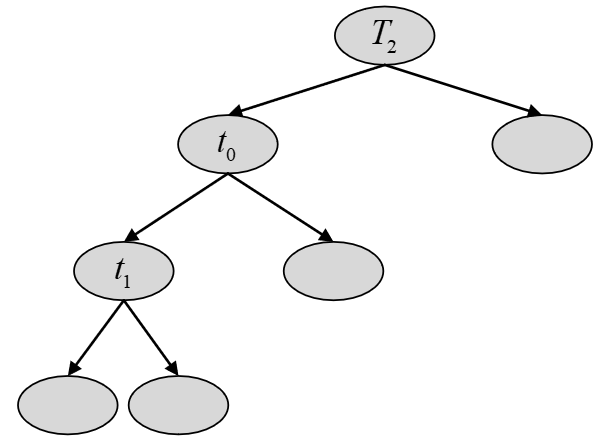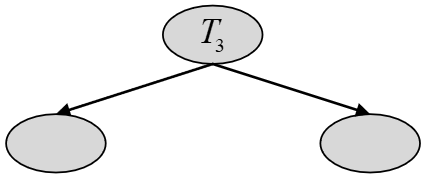xxxxxxxxxx141if __name__ == '__main__':2    from sklearn.datasets import load_iris3    from sklearn.model_selection import train_test_split4
5    data = load_iris()6    x, y = data.data, data.target7    x_train, x_test, y_train, y_test = train_test_split(x, y, test_size=0.3)8    from sklearn.tree import DecisionTreeClassifier9
10    model = DecisionTreeClassifier(criterion='gini')11    model.fit(x_train, y_train)12    print(model.score(x_test, y_test))13
14# 0.9777777

### 引用

 李航，统计机器学习，清华大学出版社